S k i l l
i n
A R I T H M E T I C

Lesson 8

# Mental Calculation

The student by now should have mastered the multiplication table.

In this Lesson, we will answer the following:

1. What is "multiplication"?
2. How do we multiply whole numbers that end in 0's?

Section 2:  The distributive property

3. What is the distributive property of multiplication?
4. How can we apply the distributive property to mental calculation?

Section 3

5. How can we multiply mentally by rounding off?

 1. What is "multiplication"? Repeated addition of a unit.

3 × 5  means  Three 5's:  5 + 5 + 5.   5 is the unit.

More precisely, multiplication is the repeated addition of one number, called the multiplicand, as many times as there are 1's in another number, called the multiplier.

Since 3 is three 1's,

3 = 1 + 1 + 1,

then

3 × 5 = 5 + 5 + 5.

3 is the multiplier, 5 is the multiplicand -- the number that is being repeatedly added.  (In the United States we write the multiplier on the left.)

As for 5 × 3, it means five 3's:

5 × 3 = 3 + 3 + 3 + 3 + 3

(We will come to the most general definition of multiplication in Lesson 26.)

The result of multiplication is called the product.

4 × 8 = 32

4 is the multiplier.  8 is the multiplicand.  32 is the product.

The order property

a)  What multiplication does this illustrate?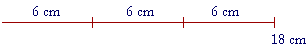Answer.   3 × 6 cm = 18 cm.  6 cm are the multiplicand.  They are being repeatedly added.

b)  What multiplication does this illustrate?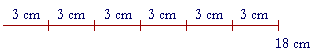Answer.   6 × 3 cm = 18 cm.  3 cm are the multiplicand.  They are being repeatedly added.

This illustrates the order property of multiplication.  If we add 3 cm six times, we will get the same number as when we add 6 cm three times.

3 × 6 cm = 6 × 3 cm

This is a property that we can prove.  And it is not dependent on knowing that 3 × 6 = 18Here again is 3 × 6 cm.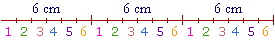Do you see 6 × 3 cm ?

Look at the 1st cm in each 6.  There are 3 cm.  Now look at the 2nd.  There's another 3.  And so on, up to the 6th in each group. There are 6 × 3 cm.   And it was not necessary to name the product(In algebra, this property of multiplication has the strange 19th century name of the commutative law.  For the indication of a formal proof, see the end of the following section.)

We now turn our attention to certain skills.

The order of any factors

When numbers are multiplied, they are called the factors of the product.

2 × 3 × 5

The factors are 2, 3, and 5.

The order of any number of factors does not matter.

2 × 3 × 5 = 5 × 2 × 3 = 5 × 3 × 2

And so on.  In each case, we will get 30.  We may group the factors as we please.

Example 1.   Multiply as easily as you can:  7 × 2 × 9 × 5

Solution.   Take advantage of factors that produce a multiple of 10:

7 × 2 × 9 × 5 = (2 × 5) × (7 × 9) = 10 × 63 = 630.

Here is another essential skill:

 2. How do we multiply whole numbers that end in 0's? 200 × 30 Ignore those final 0's and multiply the numbers that remain. Then, put back all the 0's that you ignored.

Example 2.   200 × 30 = 6000.

Ignore the final 0's and simply multiply

2 × 3 = 6

Now we ignored a total of three 0's.  Therefore we must put back those three 0's:

200 × 30 = 6000

When we ignore final 0's, we have in effect divided
by a power of 10. (Lesson 1, Question 11.) Therefore to compensate and name the right answer, we must multiply by that power -- we must add on the same number of 0's.

For a number does not change if we divide it and then multiply the quotient by the same number.

See Lesson 10.

Example 3.    9 × 20 = 180

Example 4.   Multiply  3 × \$.40  mentally.

Answer.  Ignore the decimal point for the moment, and we have

3 × 40 = 120

Now put back those two decimal places:

3 × \$.40 = \$1.20

(We will explain in the next Lesson about ignoring decimal points and then replacing them.)

Example 5.   7 × \$.80 = \$5.60

Expanding the multiplier

Since multiplication is repeated addition, we can often go from what we know to what we do not know.  Say, for example, that you do not know 12 × 8.  But Twelve 8's are composed of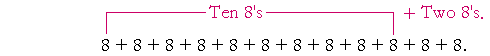10 × 8 = 80,

2 × 8 = 16.

Therefore,

12 × 8 = 80 + 16 = 96.

8 is the unit.  We are counting 8's.

Example 6.   Calculate  16 × 5  mentally by expanding 16 into its tens and ones.

 Answer.   Sixteen 5's are equal to Ten 5's  +  Six 5's: 50 + 30 = 80

Example 7.   Calculate  34 × 6  mentally by expanding 34.

Solution.   34 × 6  =  30 × 6  +  4 × 6  =  180 + 24  =  204

"Thirty-four 6's are equal to Thirty 6's plus four 6's."

Example 8.  Multiplying by 11.    11 × 45.

Multiplication by 11 is particularly easy, because 11 = 10 + 1.   Therefore, 11 × 45 is equal to 10 × 45  +  1 × 45:

450 + 45 = 495.

Example 9.   11 × 6.2 = 62 + 6.2 = 68.2

To do this or any of these problems in writing --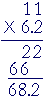-- is nothing more than a trick to get the right answer.

Example 10.   How much is 13 × 12?

Answer.  If you know that

12 × 12 = 144,

then 13 × 12 is simply one more 12:

13 × 12 = 144 + 12 = 156

Or, we could count the 12's like this:

 Thirteen 12's =  Ten 12's + Three 12's =  120 + 36 =  156.

This is the property of adding multiples of a number, which we can express it as follows:

A sum of units does not depend on the unit.

In other words, 2 units + 3 units = 5 units, no matter what the unit. 2 chairs + 3 chairs = 5 chairs. And 2 nines + 3 nines = 5 nines.

(Since this is true in arithmetic, the right-hand distributive law of algebra may be applied to arithmetic.)

How to square a number mentally

A square figure is a four-sided figure in which all the sides are equal, and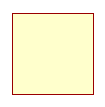all the angles are right angles.

To square a number means to multiply it by itself.

"9 squared" = 9 × 9 = 81.

To find the area of a square figure -- that is, the space enclosed by the four straight lines -- square the length of a side. (See Lesson 9.)  If each side were measured by number 6, for example, then the area would be measured by 6 × 6 = 36.

Now, say that we want to square a two-digit number such as 24, mentally.  The following geometrical arithmetic illustrates how.

Look at this square figure in which each side is 24.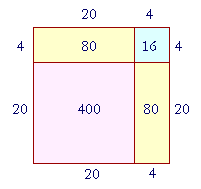Then the area of that square will be 24 × 24.  But we can break up 24 into 20 + 4.  Therefore the entire square will be composed of the following:

The square of 20, which is 400.

Two rectangles, each with area 20 × 4 = 80.

And the square of 4, which is 16.

In other words, the square of  20 + 4  is equal to

The square of 20, plus

Two times 20 × 4, plus

The square of 4.

400 + 160 + 16 = 576.

This is not a difficult mental calculation.

Example 11.   Square 52.

Solution.   52 = 50 + 2.

The square of  50 + 2  is equal to

The square of 50, plus

Two times 50 × 2, plus

The square of 2.

2500 + 200 + 4 = 2704.

Problem 1.   Square 35.

To see the answer, pass your mouse over the colored area.
To cover the answer again, click "Refresh" ("Reload").
Do the problem yourself first!

The square of  30 + 5  is equal to:

The square of 30  +  Two times 30 × 5  +  The square of 5.

900 + 300 + 25 = 1225.

Example 12.   Square 48.

Solution.   Rather than square 40 + 8, it is simpler to square 50 − 2.  In this case, the square of 50 − 2 equals

The square of 50, minus

Two times 50 × 2, plus

The square of 2.

2500 − 200 + 4 = 2304.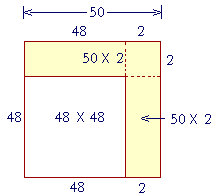The entire square is the square of 50.  It is composed of the square of 48, plus two rectangles, 50 × 2 -- which we must subtract.  But we should not subtract the square of 2 twice.  Therefore we add it at the end.

Problem 2.   Square 39.

The square of  40 − 1  is equal to:

The square of 40  −  Two times 40 × 1  +  The square of 1.

1600 − 80 + 1 = 1521.

At this point, please "turn" the page and do some Problems.

or

Continue on to the Section 2:

www.proyectosalonhogar.com